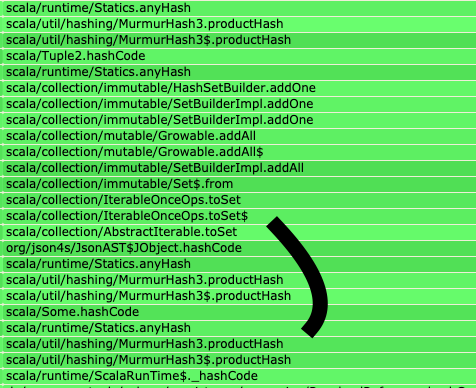## Bitten by hash codes on production

4 minute read 24 February 2021

# Hash codes can be surprising

I'd like to write about a performance issue I found out on production.

This issue is coming from change to a Scala function. That change looked totally innocent at the first place.

The code in question is about fetching JSON object from external resources. The function accepts `ids` as input and returns a `Map` where the value is an `Option[JObject]` to represent, for each `id` if a JSON object could be found or not.

I'll try to explain this with some code. I simplified the original code a lot to focus on the important port.

First some code just to fake the data structures used later:

``````case class Id(value: String)

// just to represent a JSON object
case class JObject(fields: List[(String, Any)])

def loadFromExternalServer(id: Id): Option[JObject] =
if (id.value.hashCode() % 2 == 0) // simulate finding the external resource or not
None
else
Some(JObject(List("id" -> id.value, "name" -> "a name")))
``````

### The initial implementation

The function was initially implemented like this:

``````def loadRecords(ids: Seq[Id]): Map[Id, Option[JObject]] =
ids
.map(id => (id, loadFromExternalServer(id)))
.toMap
``````

If we call this function, we will get the expected `Map`:

``````val ids = Seq(Id("id-1"), Id("id-2"), Id("id-3"), Id("id-4"), Id("id-5"))

loadRecords(ids)
// Map(Id(id-1) -> Some(JObject(List((id,id-1), (name,a name)))), Id(id-5) -> Some(JObject(List((id,id-5), (name,a name)))), Id(id-4) -> None, Id(id-3) -> Some(JObject(List((id,id-3), (name,a name)))), Id(id-2) -> None)
``````

### The change

At some point, one notices that we sometimes are calling `loadRecords` with duplicated ids. To avoid that, we changed the `Seq` to a `Set` to make sure that all ids are unique:

``````def loadRecords(ids: Set[Id]): Map[Id, Option[JObject]] =
ids
.map(id => (id, loadFromExternalServer(id)))
.toMap
``````

As you can notice, we only changed `ids: Seq[Id]` to `ids: Set[Id]` in the function signature.

The result of calling this function does not change:

``````val ids = Set(Id("id-1"), Id("id-2"), Id("id-3"), Id("id-4"), Id("id-5"))

loadRecords(ids)
// Map(Id(id-1) -> Some(JObject(List((id,id-1), (name,a name)))), Id(id-5) -> Some(JObject(List((id,id-5), (name,a name)))), Id(id-4) -> None, Id(id-3) -> Some(JObject(List((id,id-3), (name,a name)))), Id(id-2) -> None)
``````

### The impact

When looking at the servers on production, I found a strange part in one flame graph:The servers were spending a significant amount of time computing the hash codes of JSON objects.

### The explanation

It took me some time to figure out the issue.

Let's focus on this part:

``````  ids
.map(id => (id, loadFromExternalServer(id)))
``````

This piece of code produced a `Seq[(Id, Option[JObject])]` before the change. After the change, it produces a `Set[(Id, Option[JObject])]`.

For every item inserted, a `Set` must check for duplicates. It does that with the help of the hash code of each element.

After the change, `Set` was also asking for the `hashCode()` of the `JObject`, computed recursively by traversing the whole JSON object. For large objects, this computation can be costly. (At least, costly enough to make it apparent in a flame graph)

This change went unnoticed because the `hashCode()` method exists on all objects in Java.

If we had used type classes, we may have noticed this issue before. By assuming that there is no instance of `def hashCode(): Int` for `JObject` (which seems fair), the compiler would have fail.

### Avoiding intermediate collections

To avoid computing intermediate collections, we can also use the `iterator()`:

``````def loadRecords(ids: Set[Id]): Map[Id, Option[JObject]] =
ids
.iterator
.map(id => (id, loadFromExternalServer(id)))
.toMap
``````

That way, we avoid instantiating the intermediate `Seq` or `Set`.